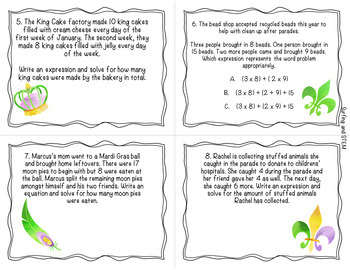Subject
Resource Type
File Type

Zip

(6 MB|24 pages)
Product Rating
Standards
• Product Description
• StandardsNEW

Mardi Gras season is here! Spice up your math block with a review and these Mardi Gras themed task cards! The fifth grade skills reviewed are fractions, operations in base ten, and operations and algebraic thinking (expressions and equations). These put a great twist on ant activity to incorporate the season and also are a great way to focus on word problems.

Solve real world problems involving division of unit fractions by non-zero whole numbers and division of whole numbers by unit fractions, e.g., by using visual fraction models and equations to represent the problem. For example, how much chocolate will each person get if 3 people share 1/2 lb of chocolate equally? How many 1/3-cup servings are in 2 cups of raisins?
Interpret division of a unit fraction by a non-zero whole number, and compute such quotients. For example, create a story context for (1/3) ÷ 4, and use a visual fraction model to show the quotient. Use the relationship between multiplication and division to explain that (1/3) ÷ 4 = 1/12 because (1/12) × 4 = 1/3.
Solve real world problems involving multiplication of fractions and mixed numbers, e.g., by using visual fraction models or equations to represent the problem.
Add, subtract, multiply, and divide decimals to hundredths, using concrete models or drawings and strategies based on place value, properties of operations, and/or the relationship between addition and subtraction; relate the strategy to a written method and explain the reasoning used.
Find whole-number quotients of whole numbers with up to four-digit dividends and two-digit divisors, using strategies based on place value, the properties of operations, and/or the relationship between multiplication and division. Illustrate and explain the calculation by using equations, rectangular arrays, and/or area models.
Total Pages
24 pages
Included
Teaching Duration
N/A
Report this Resource to TpT
Reported resources will be reviewed by our team. Report this resource to let us know if this resource violates TpT’s content guidelines.